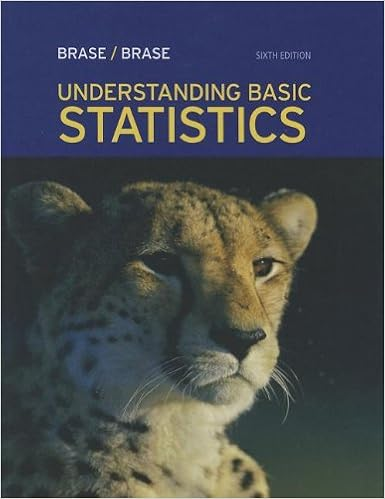1 1 point what is the problem or question to be

• 3
• 100% (5) 5 out of 5 people found this document helpful

This preview shows page 2 - 3 out of 3 pages.

We have textbook solutions for you!
The document you are viewing contains questions related to this textbook.The document you are viewing contains questions related to this textbook.
Chapter 11 / Exercise 1
Understanding Basic Statistics
Brase/BraseExpert Verified
1. (_/1 point) What is the problem or question to be solved? How fast do specify tectonic plates move/spread? 2. (_/1 point)
2. a. (_/1 point) What is the hypothesis that was tested? Note that a hypothesis
2. b (_/1 point)
3. (_/1 point) Based on your answer to the previous questions, how was the
4. (_/1 point)
5. (_/1 point) Based on the experimental result, what biases or error sources
We have textbook solutions for you!
The document you are viewing contains questions related to this textbook.The document you are viewing contains questions related to this textbook.
Chapter 11 / Exercise 1
Understanding Basic Statistics
Brase/BraseExpert Verified
•••